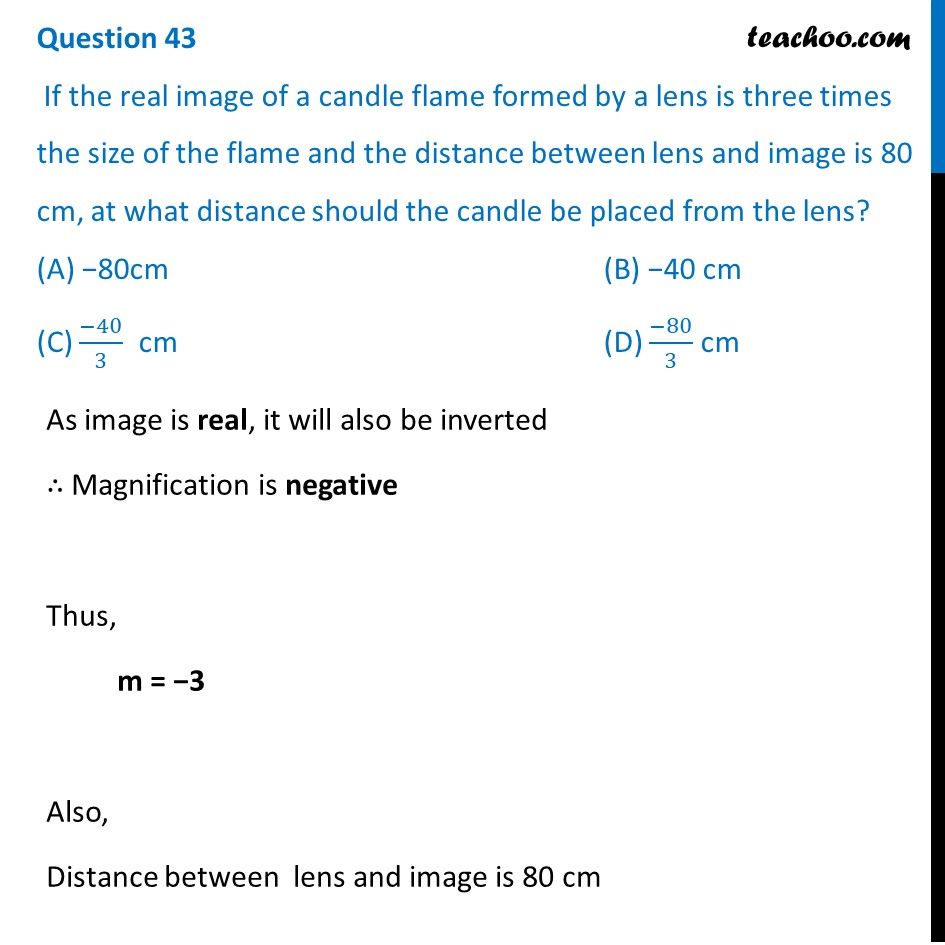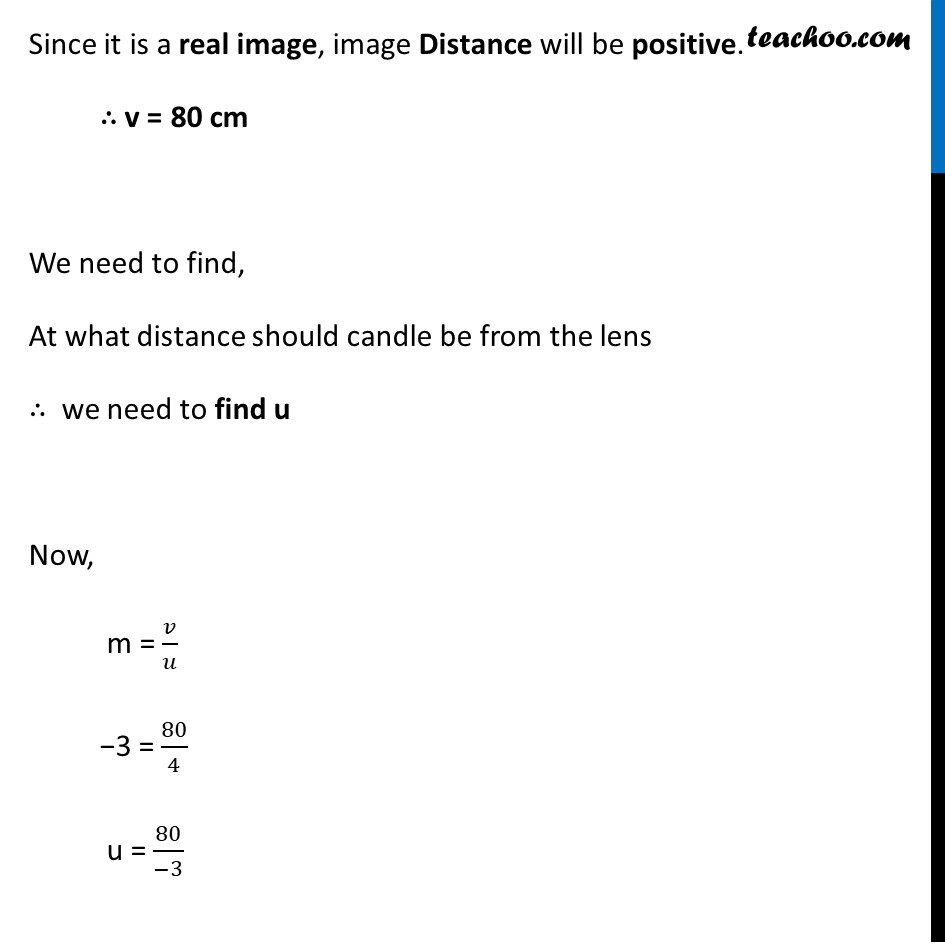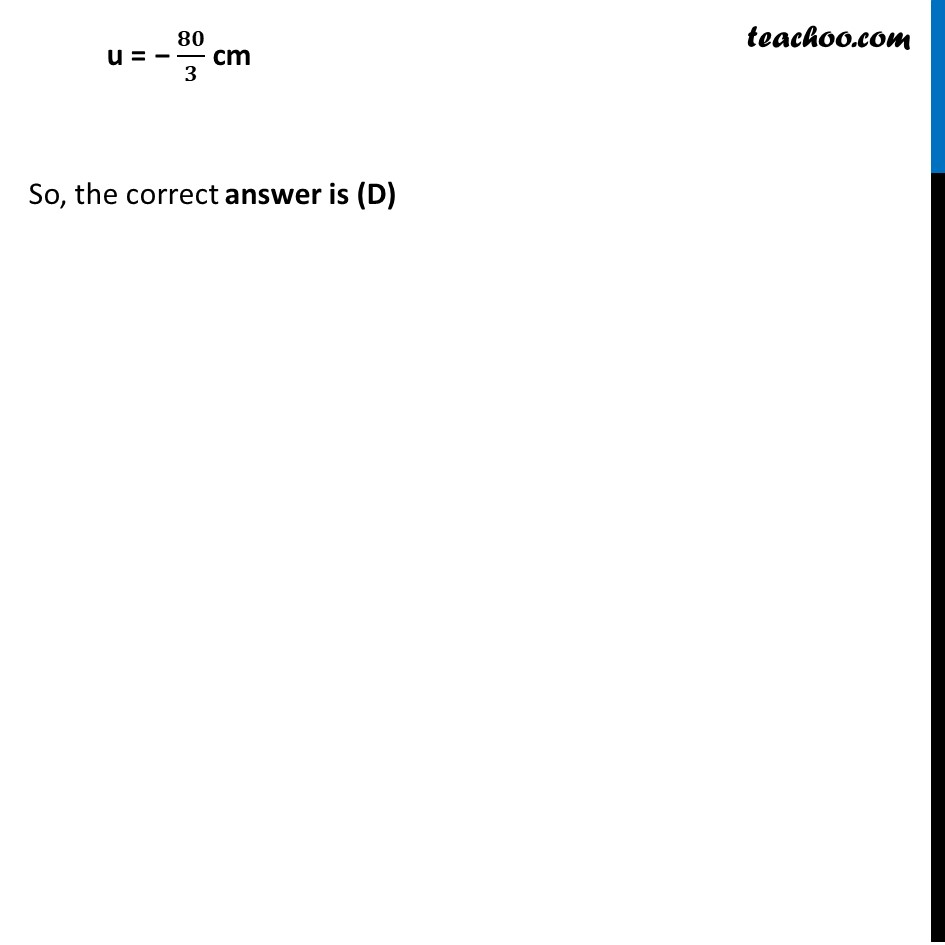Solutions - CBSE Class 10 Sample Paper for 2022 Boards - Science [MCQ]

Class 10
Solutions to CBSE Sample Paper - Science Class 10

## (D) -80/3 cmLearn in your speed, with individual attention - Teachoo Maths 1-on-1 Class

### Transcript

Question 43 If the real image of a candle flame formed by a lens is three times the size of the flame and the distance between lens and image is 80 cm, at what distance should the candle be placed from the lens? (A) -80cm (B) -40 cm (C) -40/3 cm (D) -80/3 cm As image is real, it will also be inverted ∴ Magnification is negative Thus, m = −3 Also, Distance between lens and image is 80 cm Since it is a real image, image Distance will be positive. ∴ V = 80 cm We need to find, At what distance should candle be from the lens ∴ we need to find u Now, m = 𝑉/𝑢 −3 = 80/4 u = 80/(−3) u = − 𝟖𝟎/𝟑 cm So, the correct answer is (D)